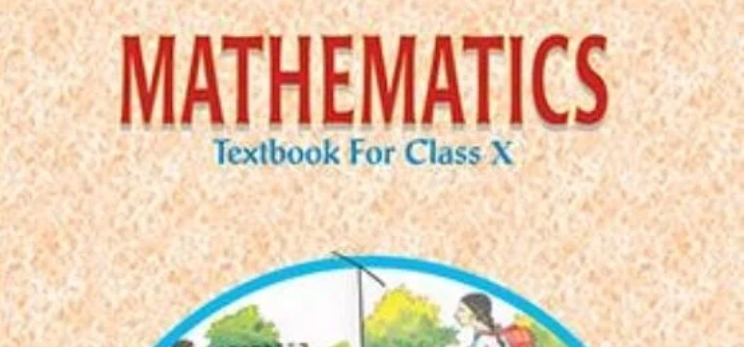## NCERT Solutions for Class 10 Maths – Latest Solutions

NCERT Solutions for Class 10 Maths are given for the students so that they can get to know the answers to the questions in case they are not able to find it. It is important for all the students who are in Class 10 currently. Here we are providing the solutions to all the chapters of NCERT Maths Class 10 Textbook for the students. They can refer to these solutions while they are solving the questions from the textbook.

## NCERT Solutions for Class 10 Maths

The students who are in class 10 and have the NCERT affiliated Maths Books can check this page to know the solutions for all the chapters of Maths book. They need to ensure that they are checking the solutions for the chapter which they intend to check.

## NCERT Solutions for Class 10 Maths

NCERT Solutions for Class 10 Maths are given below for all chapter. Select chapter number to view NCERT Solution chapter wise.

### NCERT Solutions Subject wise

We are giving the subjectwise solution of NCERT Class 10 books. They will find chapter wise solutions for each subject which can be downloaded.